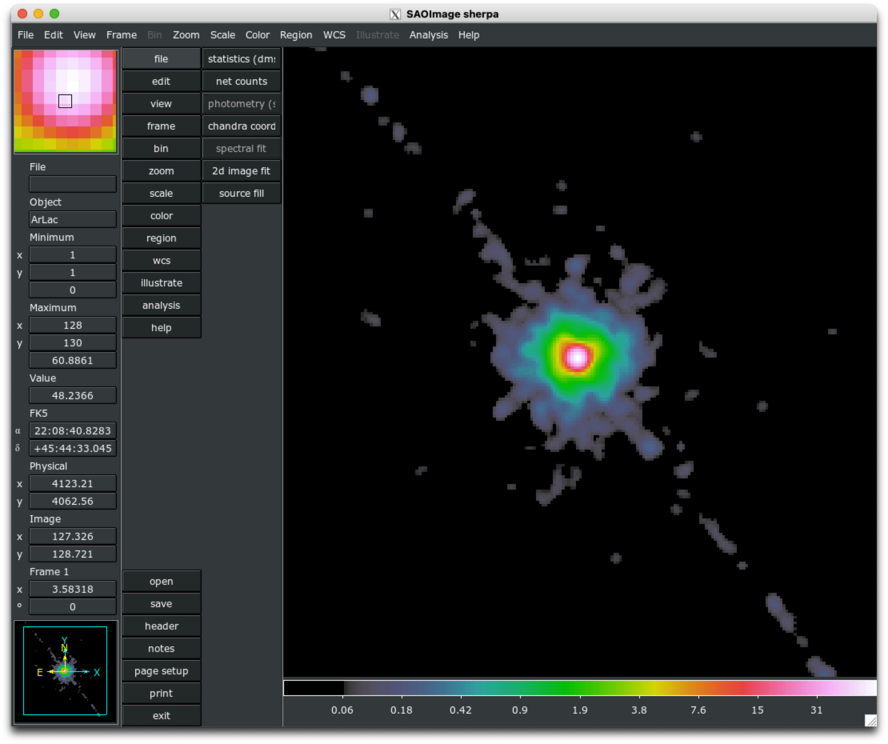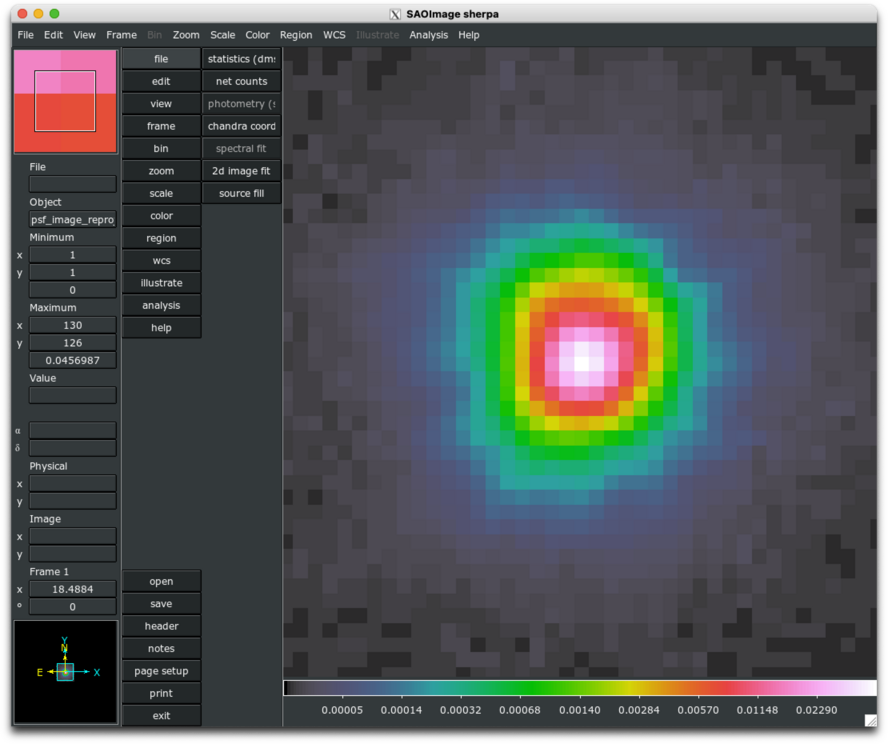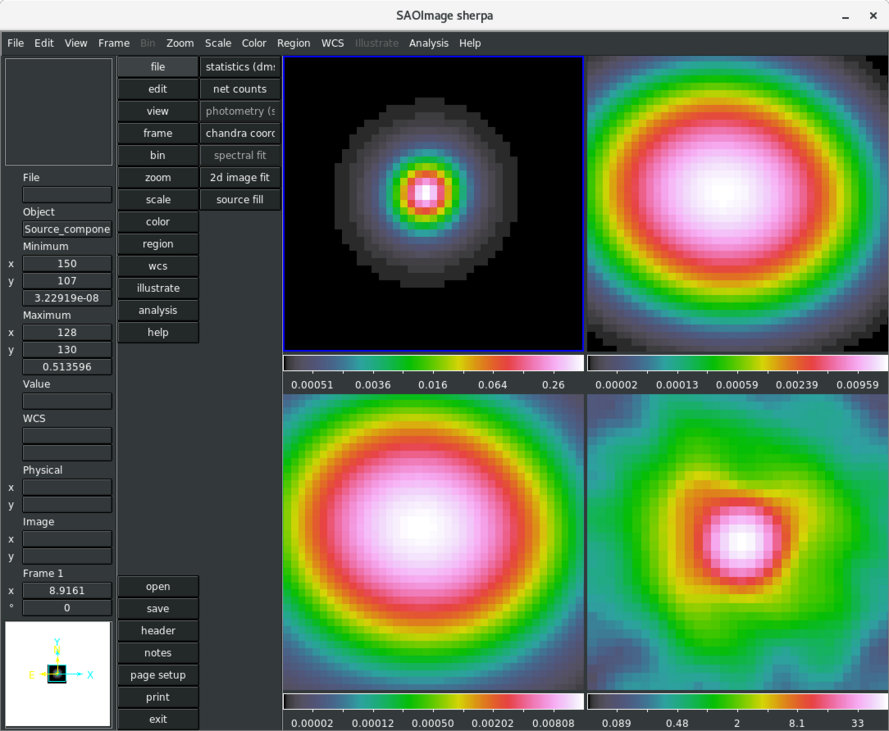# Fitting Image Data with Convolved and Unconvolved Model Components## Overview

#### Synopsis:

This thread demonstrates the use of the set_full_model functionality for manually defining a complete source model expression for fitting spatial 2-D data, where one or more components are convolved by a PSF model, and others are not. An exposure map file is input to Sherpa as a file-based exposure map model via the load_table_model function, and used in the fitting of all model components, both unconvolved extended emission and convolved point-source emission. A Point-Spread-Function (PSF) image is loaded via load_psf to be used as the convolution kernel for the point-source emission, only.

Last Update: 10 Dec 2015 - reviewed for CIAO 4.8, no content change.

## Reading and Plotting 2D FITS Data

In this thread we use 2D spatial data from the FITS data file src_image.fits, which was created by binning a Chandra events table in sky coordinates and filtering it to contain only the source region of interest—a square region of extended source emission containing a bright central point source. This data set is input to Sherpa with the load_image command, and can be visualized with the image_data or contour_data commands.

```sherpa> load_image("src_image.fits")

sherpa> image_data()
```

The image_data command opens the source data image in DS9, as shown in Figure 1, where in this example, each pixel subtends 0.492 arcseconds (a sky coordinate binning factor of 1 was used).

### Figure 1: Source data image### Figure 1: Source data image

Image of source data sent to DS9 by the Sherpa image_data function. The image has been smoothed in DS9 using a 2σ Gaussian.

The show_data command lists information available for this data set, such as the binning of the image, physical dimensions, and coordinate system.

```sherpa> show_data()
Data Set: 1
Filter:
name      = src_image.fits
x0        = Float64
x1        = Float64
y         = Float64
shape     = (513, 513)
staterror = None
syserror  = None
sky       = physical
crval    = [ 3815.5  3688.5]
crpix    = [ 0.5  0.5]
cdelt    = [ 1.  1.]
eqpos     = world
crval    = [ 116.0688   37.9087]
crpix    = [ 4096.5  4096.5]
cdelt    = [-0.0001  0.0001]
crota    = 0
epoch    = 2000
equinox  = 2000
coord     = logical
```

## Defining a Source Model

In order to define a multi-component source model expression in Sherpa containing both convolved and unconvolved components with which to fit a single data set, the model must be manually defined using the set_full_model function (as opposed to the set_source function, which automatically convolves an entire source model expression with a PSF or response model). After examining Figure 1, it is apparent that a 2D Beta model is appropriate for describing the extended component of the source emission, while a PSF-convolved 2D Gaussian model can be used to describe the central point-source component. We choose to apply an (optional) exposure map model to the full source model expression to account for a variable response across the data image, and convolve only the point-source model component by a PSF model. First, we set up the exposure map and PSF model components, and then define the full source model expression with set_full_model.

### Setting the exposure map

We define a file-based exposure map model by loading an exposure map file with the load_table_model command:

```sherpa> load_table_model("emap", "expmap.fits")
```

To display the status of the model emap, use the print() command; notice that Sherpa identifies the exposure map model as "tablemodel.emap":

```sherpa> print(emap)
tablemodel.emap
Param        Type          Value          Min          Max      Units
-----        ----          -----          ---          ---      -----
emap.ampl    thawed            1 -3.40282e+38  3.40282e+38
```

### Setting the PSF convolution model

A PSF instrument model may be established by loading an image of a PSF into Sherpa, e.g. one created with the Chandra Ray Tracer (ChaRT), or by defining a Sherpa model expression which describes the shape of the PSF. Both types of PSF models are added to the current list of models in the session using the load_psf command. In this example, we load an image containing the Chandra PSF at 1 keV, at the position of our point source on the ACIS-S3 chip.

```sherpa> load_psf("psf0", "psf_image.fits")

sherpa> print(psf0)
psfmodel.psf0
Param        Type          Value          Min          Max      Units
-----        ----          -----          ---          ---      -----
psf0.kernel  frozen psf_image.fits
psf0.norm    frozen            1            0            1
```

Normally—i.e., when set_source is used to define a source model expression—the model to be used as the PSF convolution model must be defined as such with the set_psf command; however, it is not necessary in this case since we are manually defining the full source model expression using the set_full_model functionality, as shown in the next section of this thread. To learn how to fully establish a PSF model when defining a source model with set_source, see the Sherpa thread Accounting for PSF Effects in 2D Image Fitting.

While it is not strictly necessary to run the set_psf command in this context, it must be run in order to utilize most of the PSF-specific Sherpa commands, e.g. the image_psf command for viewing the PSF image in DS9. Below, we go ahead and run set_psf in order to visualize the PSF model:

```sherpa> set_psf(psf0)

sherpa> image_psf()
```

The PSF image is shown in Figure 2.

### Figure 2: PSF image### Figure 2: PSF image

Running the set_psf command also allows us to take advantage of the show_psf and show_kernel commands, which offer more information than "print(psf0)":

```sherpa> show_kernel()

PSF Kernel: 1
psfmodel.psf0
Param        Type          Value          Min          Max      Units
-----        ----          -----          ---          ---      -----
psf0.kernel  frozen psf_image.fits
psf0.size    frozen   (256, 256)   (256, 256)   (256, 256)
psf0.center  frozen   (128, 128)   (128, 128)   (128, 128)
psf0.norm    frozen            1            0            1

sherpa> show_psf()

PSF Model: 1
name      = psf_image.fits
x0        = Float64
x1        = Float64
y         = Float64
shape     = (256, 256)
staterror = None
syserror  = None
sky       = physical
crval    = [ 3944.  3817.]
crpix    = [ 0.5  0.5]
cdelt    = [ 1.  1.]
eqpos     = world
crval    = [ 116.0688   37.9087]
crpix    = [ 4096.5  4096.5]
cdelt    = [-0.0001  0.0001]
crota    = 0
epoch    = 2000
equinox  = 2000
coord     = logical

```

### Defining a manual source model with set_full_model

We define the complete source model expression using set_full_model as follows, where we also include a Sherpa const2d model component to describe the constant background level contributing to the total emission:

```sherpa> set_full_model(emap*(const2d.bgnd + beta2d.ext + psf0(gauss2d.ps)))
```

In a set_full_model expression, any response or PSF convolution models which should be applied to one or more individual model components must be explicitly defined—in this example, the PSF convolution model named "psf0" is applied only to the 2D Gaussian model component used to describe the point-source emission.

We can print the default model parameter values for the defined model using show_model:

```sherpa> show_model()
PSF Model: 1
name      = psf_image.fits
x0        = Float64
x1        = Float64
y         = Float64
shape     = (256, 256)
staterror = None
syserror  = None
sky       = physical
crval    = [ 3944.  3817.]
crpix    = [ 0.5  0.5]
cdelt    = [ 1.  1.]
eqpos     = world
crval    = [ 116.0688   37.9087]
crpix    = [ 4096.5  4096.5]
cdelt    = [-0.0001  0.0001]
crota    = 0
epoch    = 2000
equinox  = 2000
coord     = logical

Model: 1
(tablemodel.emap * ((const2d.bgnd + beta2d.ext) + psfmodel.psf0(gauss2d.ps)))
Param        Type          Value          Min          Max      Units
-----        ----          -----          ---          ---      -----
emap.ampl    thawed            1 -3.40282e+38  3.40282e+38
bgnd.c0      thawed            1            0  3.40282e+38
ext.r0       thawed           10  1.17549e-38  3.40282e+38
ext.xpos     thawed            0 -3.40282e+38  3.40282e+38
ext.ypos     thawed            0 -3.40282e+38  3.40282e+38
ext.ellip    frozen            0            0        0.999
ext.theta    frozen            0     -6.28319      6.28319    radians
ext.ampl     thawed            1 -3.40282e+38  3.40282e+38
ext.alpha    thawed            1          -10           10
ps.fwhm      thawed           10  1.17549e-38  3.40282e+38
ps.xpos      thawed            0 -3.40282e+38  3.40282e+38
ps.ypos      thawed            0 -3.40282e+38  3.40282e+38
ps.ellip     frozen            0            0        0.999
ps.theta     frozen            0     -6.28319      6.28319    radians
ps.ampl      thawed            1 -3.40282e+38  3.40282e+38
```

Before fitting the model to the source, we can set some initial model parameter values:

```sherpa> ext.r0 = 7.6
sherpa> ext.xpos = 4072
sherpa> ext.ypos = 3945
sherpa> ext.ellip = 0.3
sherpa> ext.theta = 2.4
sherpa> ext.ampl = 0.42
sherpa> ext.alpha = 0.94

sherpa> ps.xpos = 4072
sherpa> ps.ypos = 3945
sherpa> ps.fwhm = 1.
sherpa> ps.ampl = 1497
sherpa> bgnd.c0 = 0.002

sherpa> thaw(ext.ellip, ext.theta, ps.ellip, ps.theta)

sherpa> freeze(emap.ampl)
```

The updated model parameters can be viewed again with show_model:

```sherpa> show_model()

{...psf model information shown above...}

Model: 1
(tablemodel.emap * ((const2d.bgnd + beta2d.ext) + psfmodel.psf0(gauss2d.ps)))
Param        Type          Value          Min          Max      Units
-----        ----          -----          ---          ---      -----
emap.ampl    frozen            1 -3.40282e+38  3.40282e+38
bgnd.c0      thawed        0.002            0  3.40282e+38
ext.r0       thawed          7.6  1.17549e-38  3.40282e+38
ext.xpos     thawed         4072 -3.40282e+38  3.40282e+38
ext.ypos     thawed         3945 -3.40282e+38  3.40282e+38
ext.ellip    thawed          0.3            0        0.999
ext.theta    thawed          2.4     -6.28319      6.28319    radians
ext.ampl     thawed         0.42 -3.40282e+38  3.40282e+38
ext.alpha    thawed         0.94          -10           10
ps.fwhm      thawed            1  1.17549e-38  3.40282e+38
ps.xpos      thawed         4072 -3.40282e+38  3.40282e+38
ps.ypos      thawed         3945 -3.40282e+38  3.40282e+38
ps.ellip     thawed            0            0        0.999
ps.theta     thawed            0     -6.28319      6.28319    radians
ps.ampl      thawed       2018.5 -3.40282e+38  3.40282e+38
```

## Fitting the model

We can now fit the manually defined model to the data in the usual way using the fit command. In this example, we decide to fit with the Sherpa C-Stat statistic and the Nelder-Mead optimization method, which we specify using the set_stat and set_method commands:

```sherpa> show_stat()
Statistic: Chi2Gehrels
Chi Squared with Gehrels variance

sherpa> show_method()
Optimization Method: LevMar
name    = levmar
ftol    = 1.19209289551e-07
xtol    = 1.19209289551e-07
gtol    = 1.19209289551e-07
maxfev  = None
epsfcn  = 1.19209289551e-07
factor  = 100.0
verbose = 0

sherpa> set_stat("cstat")

sherpa> fit()
Dataset               = 1
Statistic             = cstat
Initial fit statistic = 3.47915e+06
Final fit statistic   = 168747 at function evaluation 715
Data points           = 263169
Degrees of freedom    = 263155
Probability [Q-value] = 1
Reduced statistic     = 0.641246
Change in statistic   = 3.3104e+06
bgnd.c0        0.000434043
ext.r0         8.60185
ext.xpos       4072.55
ext.ypos       3944.9
ext.ellip      0.488371
ext.theta      3.16468
ext.ampl       0.973904
ext.alpha      3.85123
ps.fwhm        1.62618
ps.xpos        4072.58
ps.ypos        3945.54
ps.ellip       0.649299
ps.theta       1.82501
ps.ampl        1497.42
```

Normally, the image_model, image_fit, and image_resid commands can be used to display the model and fit residuals images, but these commands are not compatible with the set_full_model functionality. However, we can use the image_source_component command to separately plot each of the unconvolved model components contributing to the fit of the source emission:

```sherpa> ext.xpos = 257
sherpa> ps.xpos =  257
sherpa> ext.ypos = 235
sherpa> ps.ypos =  235

sherpa> image_source_component(ext)
sherpa> image_source_component(ps, tile=True, newframe=True)
sherpa> image_source_component(psf0(ps), tile=True, newframe=True)
sherpa> image_data(tile=True, newframe=True)
```

These commands create the images displayed in Figure 3.

### Figure 3: Images of individual, fitted model components### Figure 3: Images of individual, fitted model components

DS9 display of images of the individual, fitted model components contributing to the full model description of the extended and point-source emission (some images have different scale and zoom settings for visual enhancement). In the upper-left corner of the display is the 2D Beta model component fitted to the extended source emission; in the upper-right is the unconvolved 2D Gaussian component used to describe the central point source emission; in the lower-left is the PSF-convolved 2D Gaussian component; and in the lower-right is the smoothed data image for comparison.

There are several things to note about the series of commands issued above for creating the display in Figure 3. First is that, normally, the image_model_component command is used to plot convolved model components, where the PSF or response model associated with a given model component is automatically applied. Since we have defined our source model manually with set_full_model, we must also ensure that we manually convolve source model components within the image_source_model expression, as shown above for the PSF-convolved point-source component of the model. Second, before running the image_source_component commands, the (fitted) (x,y) center position of the individual source model components in physical coordinates had to be adjusted to coincide with the center of the data image in image coordinates, for proper display in the DS9 frame—so approximately (4072, 3945) → (257,253).

## Saving a Sherpa Session

To save this Sherpa session in order to return to the analysis at a later point, the following commands may be used:

```sherpa> save("manual_fit.save")

sherpa> save_all("manual_fit.ascii")
```

The save function records all the information about the current session to the binary file manual_fit.save, and the save_all function records the session settings to an editable ASCII file.

To restore the session that was saved to the binary file manual_fit.save or ASCII file manual_fit.ascii:

```sherpa> restore("manual_fit.save")

sherpa> execfile("manual_fit.ascii")
```

## Scripting It

The file fit.py is a Python script which performs the primary commands used above; it can be executed by typing execfile("fit.py") on the Sherpa command line.

The Sherpa script command may be used to save everything typed on the command line in a Sherpa session:

```sherpa> script(filename="sherpa.log", clobber=False)
```

(Note that restoring a Sherpa session from such a file could be problematic since it may include syntax errors, unwanted fitting trials, etcetera.)

The CXC is committed to helping Sherpa users transition to new syntax as smoothly as possible. If you have existing Sherpa scripts or save files, submit them to us via the CXC Helpdesk and we will provide the CIAO/Sherpa 4.8 syntax to you.

## Summary

This thread is complete, so we can exit the Sherpa session:

```sherpa> quit
```

## History

 10 Jan 2010 original version 06 Jan 2012 reviewed for CIAO 4.4 (no changes) 13 Dec 2012 reviewed for CIAO 4.5 (no changes) 06 Dec 2013 reviewed for CIAO 4.6: typos fixed 07 Apr 2015 reviewed for CIAO 4.7, no content change. 10 Dec 2015 reviewed for CIAO 4.8, no content change.